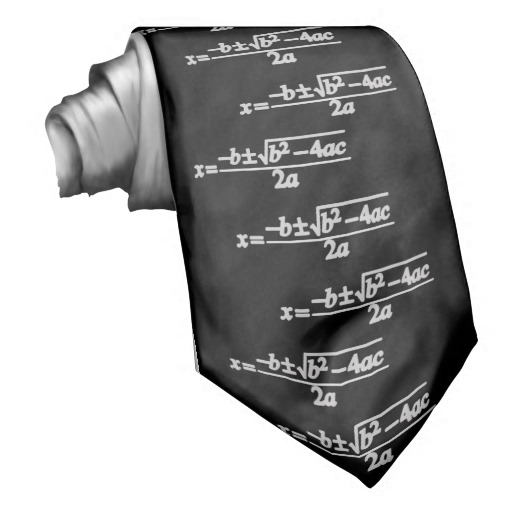Fun With Quadratic Roots.....

Algebra Level 3Let $\alpha$ and $\beta$ be the roots of the quadratic equation, $x^{2}-4x+5=0$.

What is the value of,

$\alpha^{3}+\alpha^{4}\beta^{2}+\alpha^{2}\beta^{4}+\beta^{3}?$

×